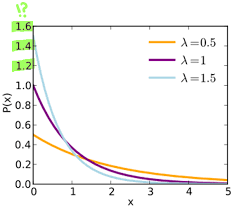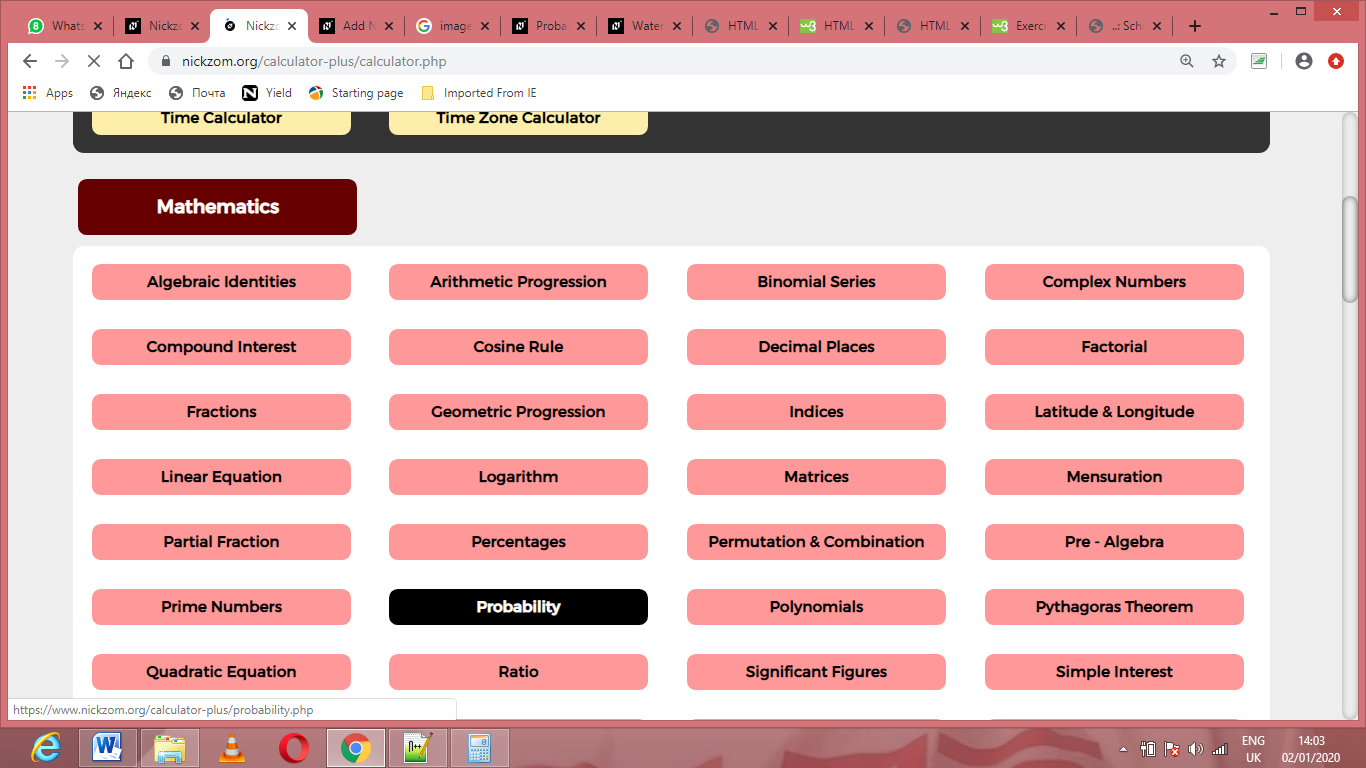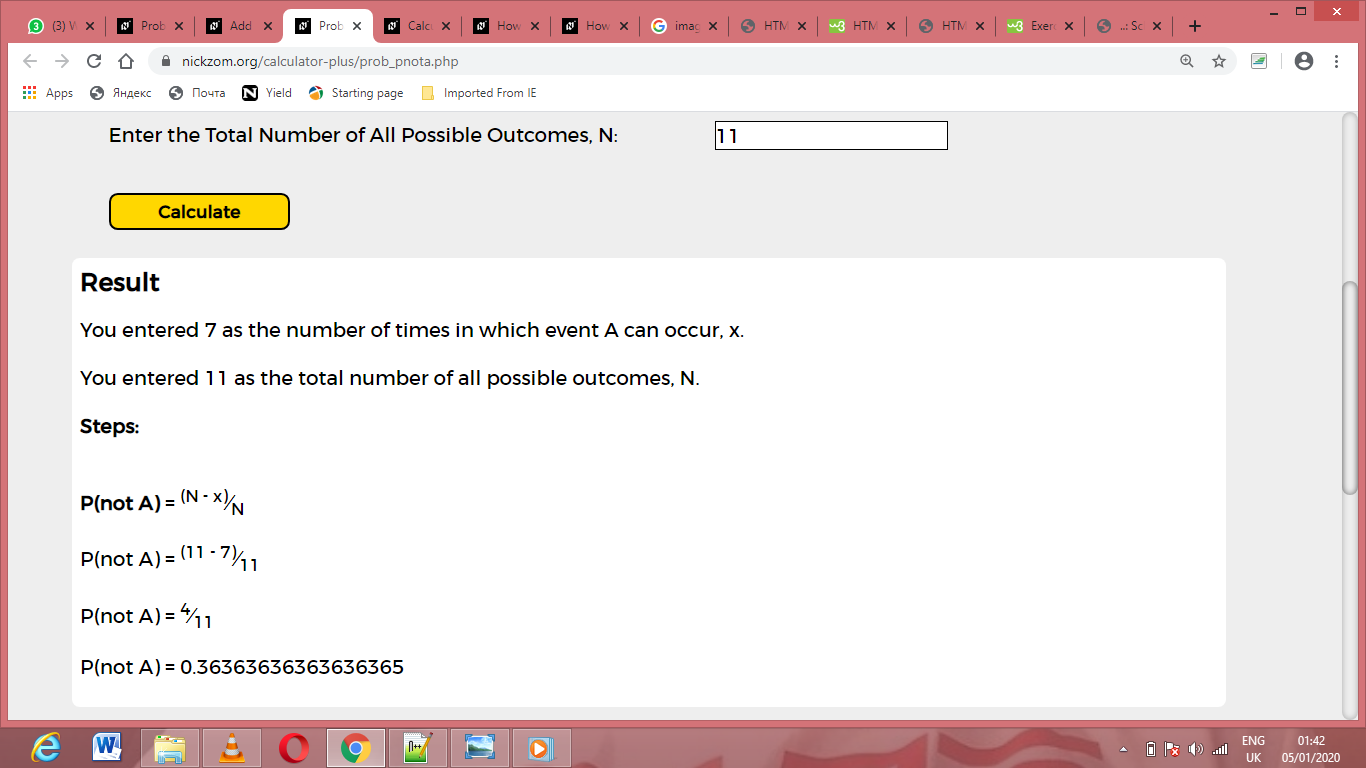# How to Calculate and Solve for P(not A) | ProbabilityThe image above represents P(not A).

To compute for P(not A), two essential parameters are needed and these parameters are Number of Times in Which Event A Can Occur (x) and Total Number of All Possible Outcomes (N).

The formula for calculating P(not A):

P(not A) = (N – x)N

Where;

x = Number of Times in Which Event A can Occur
N = Total Number of All Possible Outcomes

Let’s solve an example;
Find the P(not A) when the number of times in which event A can occur is 7 and the total number of all possible outcomes is 11.

This implies that;

x = Number of Times in Which Event A can Occur = 7
N = Total Number of All Possible Outcomes = 11

P(not A) = (N – x) N
P(not A) = (11 – 7) 11
P(not A) = 4 11
P(not A) = 0.36

Therefore, the P(not A) is 0.36.

Nickzom Calculator – The Calculator Encyclopedia is capable of calculating the P(not A).

To get the answer and workings of the P(not A) using the Nickzom Calculator – The Calculator Encyclopedia. First, you need to obtain the app.

You can get this app via any of these means:

To get access to the professional version via web, you need to register and subscribe for NGN 1,500 per annum to have utter access to all functionalities.
You can also try the demo version via https://www.nickzom.org/calculator

Apple (Paid) – https://itunes.apple.com/us/app/nickzom-calculator/id1331162702?mt=8
Once, you have obtained the calculator encyclopedia app, proceed to the Calculator Map, then click on Probability under Mathematics.Now, Click on P(not A) under ProbabilityThe screenshot below displays the page or activity to enter your values, to get the answer for the P(not A) according to the respective parameters which are the Number of Times in Which Event A Can Occur (x) and Total Number of All Possible Outcomes (N).Now, enter the values appropriately and accordingly for the parameters as required by the Number of Times in Which Event A Can Occur (x) is 7 and Total Number of All Possible Outcomes (N) is 11.Finally, Click on CalculateAs you can see from the screenshot above, Nickzom Calculator– The Calculator Encyclopedia solves for the P(not A) and presents the formula, workings and steps too.